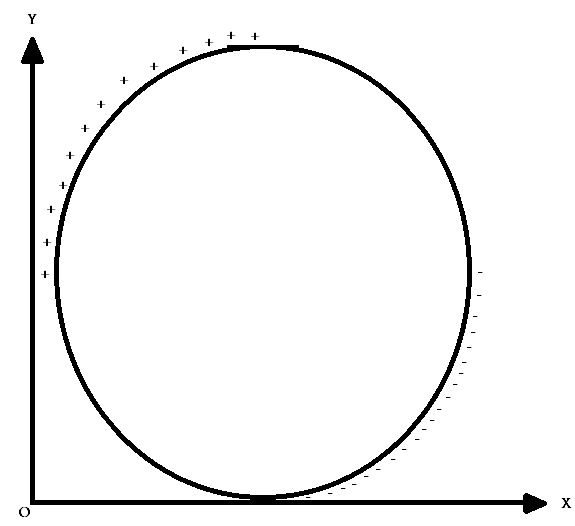# Charged Ring Rolling!

A non-conducting ring of mass $m$ and radius $R$, the charge per unit length $\lambda$ is shown in figure. It is then placed on a rough non-conducting horizontal plane. At time $t=0$, a uniform electric field $\vec{E} = E_0 \hat{i}$ is switched on and the ring starts rolling without sliding. Find the frictional force $(f)$acting on the ring.

Given - $|E_0| = 3 , R = 4m , |\lambda| = 6$

Find the value of $\sqrt{\frac{f}{2R}}$.×

Problem Loading...

Note Loading...

Set Loading...